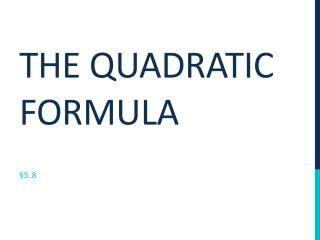DownloadDownload PresentationTélécharger la présentation- - - - - - - - - - - - - - - - - - - - - - - - - - - E N D - - - - - - - - - - - - - - - - - - - - - - - - - - -
##### Presentation Transcript

1. The quadratic formula • A quadratic equation in standard form ax2 + bx + c = 0 can be solved with:

3. Example 1: using the quadratic formula The Quadratic Formula! • Step 1) Write in standard form. • Step 2) Find the values of a, b, and c. • Step 3) Use the Quadratic Formula. • Step 4) Simplify.

4. Example 2: using the quadratic formula The Quadratic Formula! • Step 1) Write in standard form. • Step 2) Find the values of a, b, and c. • Step 3) Use the Quadratic Formula. • Step 4) Simplify.

5. Example 3: using the quadratic formula The Quadratic Formula! • Step 1) Write in standard form. • Step 2) Find the values of a, b, and c. • Step 3) Use the Quadratic Formula. • Step 4) Simplify.

6. Using the discriminant • Quadratic equations can have real or complex solutions. You can determine the type and number of solutions by finding the discriminant. • The discriminant of a quadratic equation in the form ax2 + bx+ c = 0 is the value of the expression b2 – 4ac. Discriminant

7. Is this possible? If so, what are the possible answers? Using the discriminant

8. Example 5: using the discriminant • Determine the type and number of solutions.

9. CLASSWORK 1) x2 – 4x + 3 = 0 2) 3x2 + 2x – 1 = 0 3) x2 = 3x – 1 4) 8x2 – 2x – 3 = 0